# P/V Ratio Formula

P/V Ratio Formula-

PROFIT-VOLUME RATIO (P/V Ratio) – Profit volume ratio refers to a ratio which establishes the relationship between contribution and sales.

This ratio 1s expressed as a percentage.

It is known as the P/V ratio, High P/V ratio indicates a high print provided there is no change in fixed cost.

The higher the contribution is, the greater wi be the P/V ratio.

A business can raise the P/V ratio by increasing selling price, optimum use of machines and men, increasing the efficiency of workers, etc.

This ratio is also known as Marginal Income Ratio, Contributions/Sales Ratio or, Variable Profit Ratio.

## Importance of P/V Ratio-

Profit volume ratio is a very important ratio. It helps in the calculation of many things such as…

1.To ascertain the variable cost at given sale

(ii) To calculate the Break-even pointii) To ascertain contribution at a given sale

Contribution= Sales × P/V Ratio

(iv) To take decisions in many areas

(v) To decide sales volume to achieve the desired profit

(vi) To have a comparative study of firms or products etc.

## Measures to Improve P/V Ratio-

1.By reducing variable costs

2.By increasing the selling price

3. By increasing the selling price at a rate higher than the variable costs.

4.By selling more products with higher PIN ratio.

### Also read : Golden Rules Of Accounting

P/V Ratio Formula-

### 1.When  the contribution and selling price are given in the question-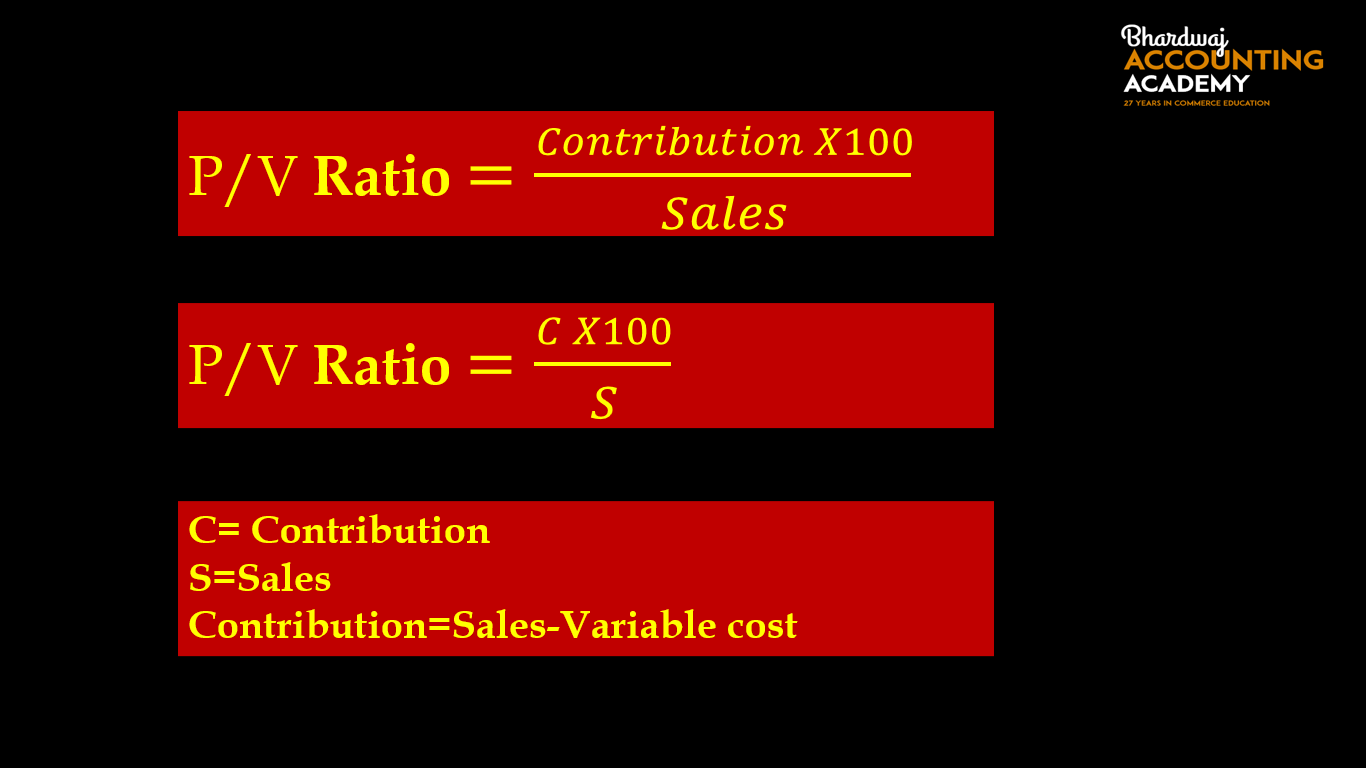The difference between selling price and variable cost is called contribution.

Or

Contribution= Fixed Cost + Profit

Or

Contribution= Fixed Cost – Loss

Or

Contribution= Sales × P/V Ratio

### 1.Example–

Find P/V Ratio from the following informations-

Selling price per unit : Rs. 200

Variable cost per unit: Rs. 120

Fixed Cost :Rs. 5,00,000

Solution-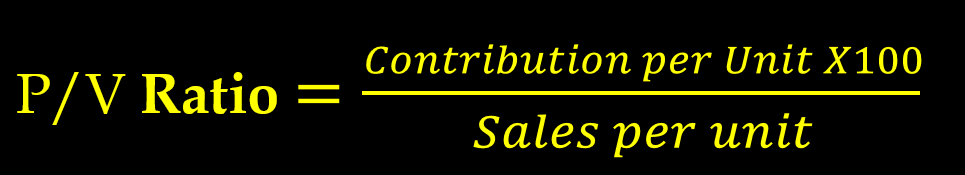Selling price per unit : Rs. 200

Variable cost per unit: Rs. 120

Contribution Per unit = 200-120

Contribution Per unit = 80

P/V Ratio= 80×100/200

P/V Ratio=40 %

2.Example

Find P/V Ratio from the following informations-

Selling price per unit : Rs. 500

Variable cost per unit: Rs. 400

Fixed Cost :Rs. 4,00,000

P/V Ratio Formula-

### 2.When   profit and sales for two financial years is  given in the question-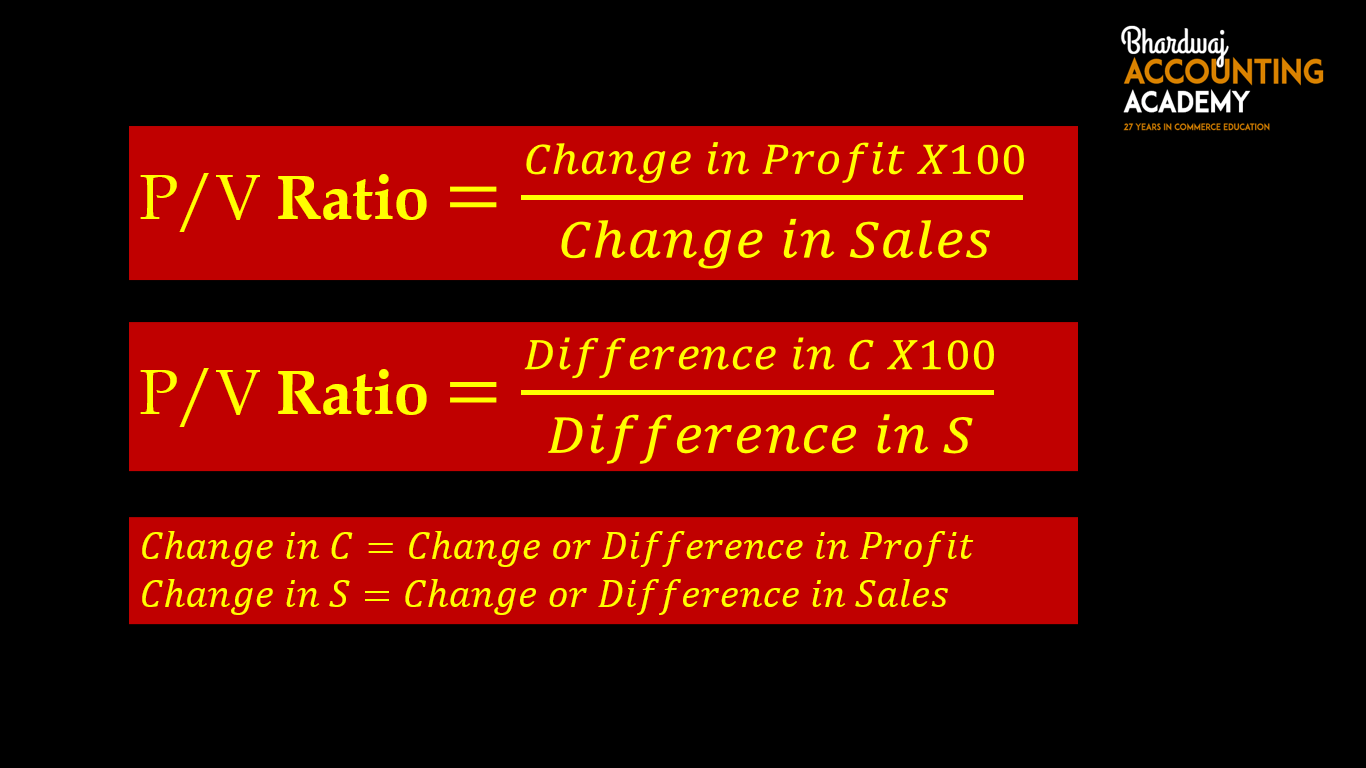### 3.Example– Find P/V Ratio from the following informations-

Sales :

2020-  Rs. 20,00,000

2021-  Rs. 24,00,000

Profit :

2020-  Rs. 3,00,000

2021-  Rs. 4,60,000

Solution-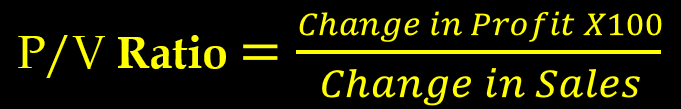Change in profit= 4,60,000-3,00,000

Change in profit=1,60,000

Change in Sales = 24,00,000-20,00,000

Change in Sales =4,00,000

P/V Ratio= 1,60,000 x 100/4,00,000

P/V Ratio=40 %

4.Example– Find P/V Ratio from the following informations-

Sales :

2020-  Rs. 50,00,000

2021-  Rs. 70,00,000

Profit :

2020-  Rs. 5,00,000

2021-  Rs. 6,80,000

P/V Ratio Formula-

### 3.When Profit, Fixed Cost and sales  is  given in the question-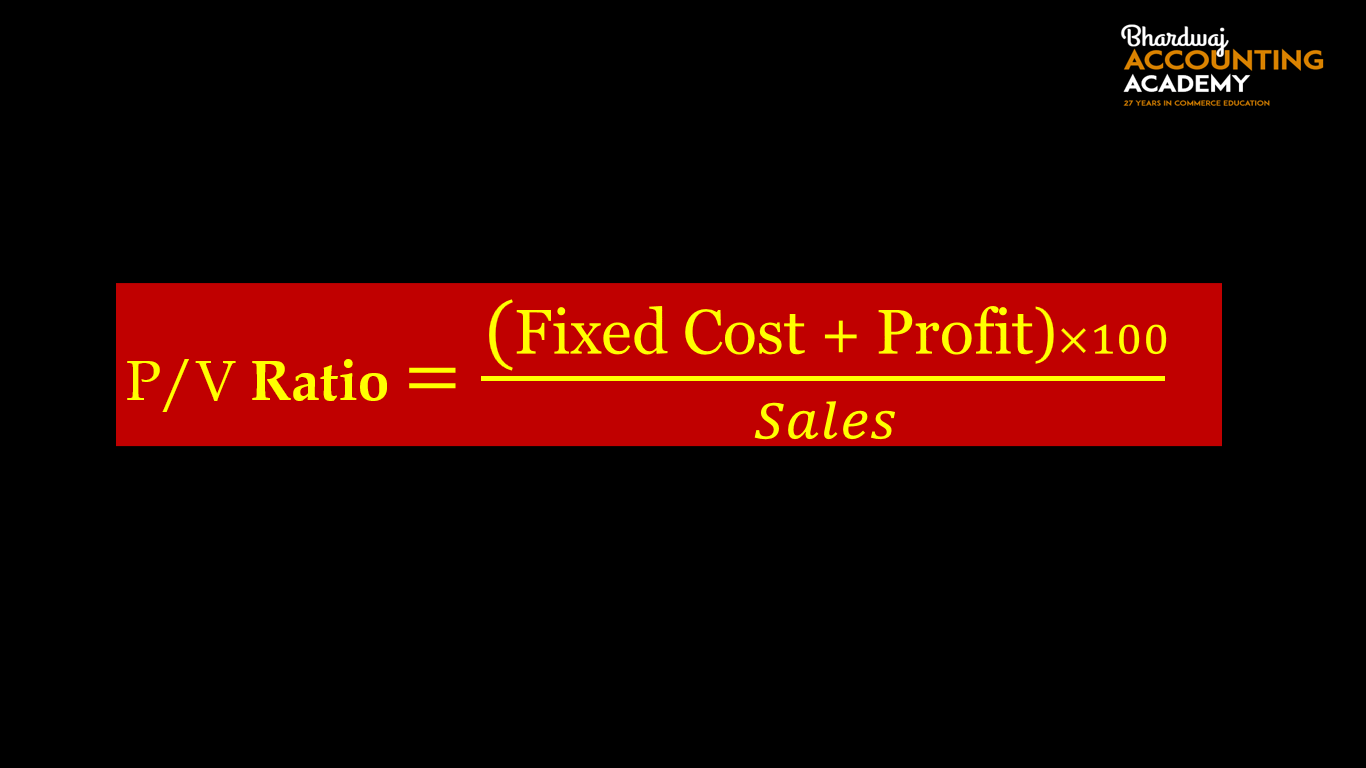### 5.Example–

Find P/V Ratio from the following informations-

Sales : Rs. 10,00,000

Profit : Rs. 2,00,000

Fixed Cost :Rs. 1,00,000

Solution-P/V Ratio= (1,00,000+2,00,000) × 100/10,00,000

P/V Ratio=3,00,000× 100/10,00,000

P/V Ratio=30 %

6.Example

Find P/V Ratio from the following informations-

Sales : Rs. 5,00,000

Profit : Rs. 1,00,000

Fixed Cost :Rs. 80,000

P/V Ratio Formula-

### 4.When Loss, Fixed Cost and sales  is  given in the question-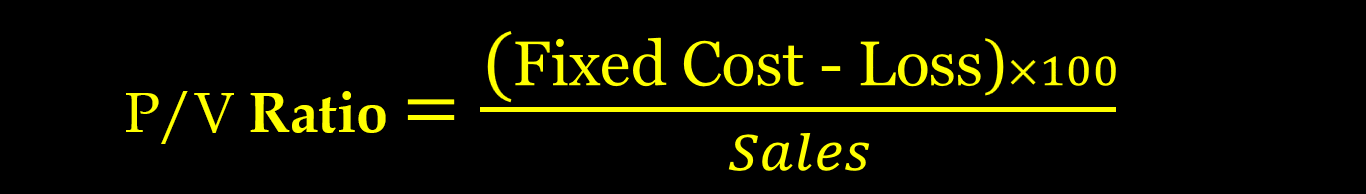### 7.Example–

Find P/V Ratio from the following informations-

Sales : Rs. 10,00,000

Loss : Rs. 2,00,000

Fixed Cost :Rs. 5,00,000

Solution-P/V Ratio= (5,00,000-2,00,000) × 100/10,00,000

P/V Ratio=3,00,000× 100/10,00,000

P/V Ratio=30 %

8.Example

Find P/V Ratio from the following informations-

Sales : Rs. 5,00,000

Loss : Rs. 50,000

Fixed Cost :Rs. 2,00,000

### Also read : REVALUATION ACCOUNT/ PROFIT & LOSS ADJUSTMENT ACCOUNT

error: Alert: Content is protected !!